Courses
Courses for Kids
Free study material
Free LIVE classes
More

# An Easy Way to Convert Fraction to DecimalLIVE
Join Vedantu’s FREE Mastercalss

## Learn How to Convert Fractions into Decimals Easily

Can you determine what percentage of a cake is pastry? And is a rupee's decimal component the paisa? You'll experience lots of examples of fractions and decimals in usage every day. Let's learn about their characteristics and mathematical operations,such as multiplication and division, in the following part.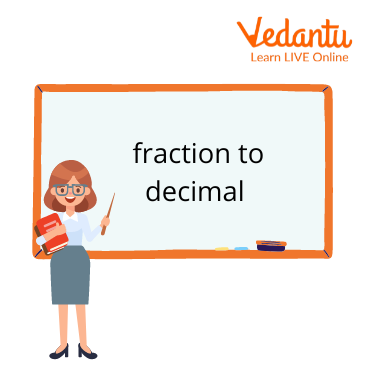Fraction to Decimal

## An Introduction to Fractions and Decimals

The process of changing a number expressed as p/q, where p and q are whole numbers and q is not equal to zero, into a decimal form can be described as the process of either changing the denominator to a power of 10 or using the long division method. In this post, we will examine various methods for converting fractions to decimals.

## Let’s Convert Fraction to Decimal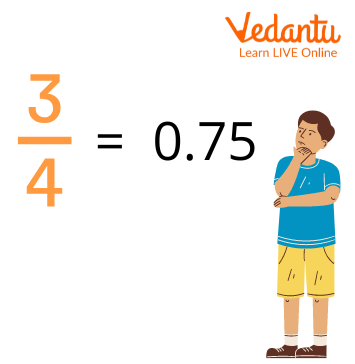Convert Fraction to Decimal

By applying a few simple procedures, you may quickly convert a fraction to a decimal:

1. ### Using a calculator

Using a calculator, divide the numerator by the denominator to convert a fraction to a decimal. The value of the fraction expressed as a decimal number will be the outcome.

1. ### Not Using a Calculator

Long division is used to solve the problem.

## How to Convert Fraction to a Decimal?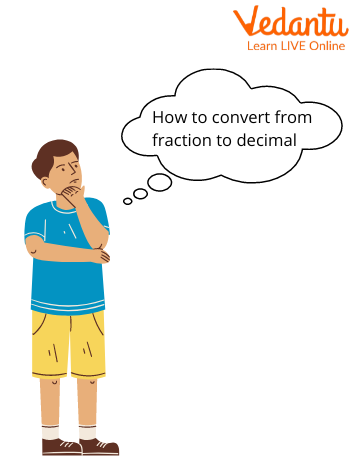How to Convert Fraction to Decimal

The following are two ways to convert a fraction to a decimal:

• Method of long division

• By changing the fraction's denominator to a power of 10

## Long Division to Convert a Fraction to a Decimal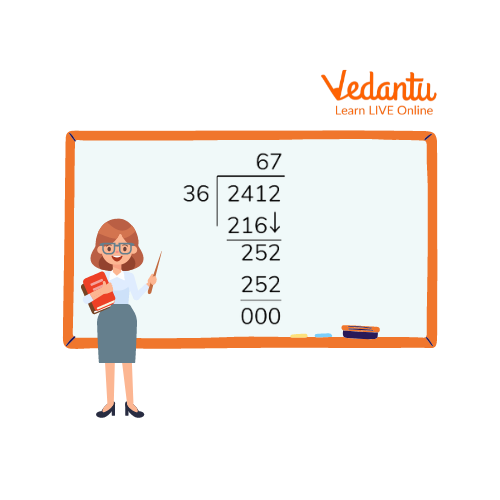Long Division to Convert Fraction to Decimal

We utilise the long division method to change a number from its fractional form, p/q, into its decimal form. In this situation, we divide the denominator by the numerator.

Following are fraction to decimal steps-

• Put 4/19 in decimal form.1.First, think of the fraction 4/19 as having a dividend of 4 and a divisor of 19 in the denominator.

• After putting the decimal (.), multiply 19 by another integer such that the result is less than or equal to 40.

• The remaining number is 2, and the digit that appears in the quotient is 2.

• In order for the result to be less than or equal to 20, multiply 19 by a number.

• Now that the quotient has an additional digit of 1, making it 0.21, the remaining number is 1.

• Continue in this manner until the quotient has at least three decimal places or 0 as the remainder.

## Change the Numerator to Convert a Fraction to a Decimal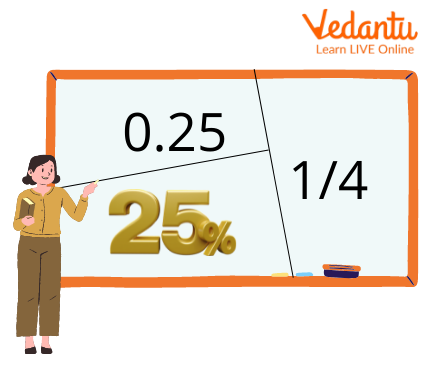Change Numerator to Convert Fraction to Decimal

The following are the fraction to decimal steps using the denominator approach.

• Decimalise 7/8, for example.

• To get a power of 10 in the denominator, we must first come up with a number that we may multiply the denominator and numerator by.

• The denominator, in this case, is 8. (125 divided by 8) = 1000.

• Now divide the denominator by the same number as the numerator, which is 125. By dividing the fraction's numerator by 125, we arrive at 7 x125 = 875.

• Once the multiplication procedure is complete, we get a denominator that is a power of 10, or 875/1000. Put a decimal point before the number of places in the denominator that are equivalent to zeros.

• So, 875/1000 equals 0.875.

## Solved ExamplesExample Time

Following is an example of how to turn a remainder into a decimal-

1. Suppose you are to divide 15 by 8.

Solution: Now 15/8 gives a quotient of 1 and a remainder of 7.

The remainder of 7 is converted into a decimal as: =(7/8)*100 = 87.5/100 = 0.875.

So the result of 15/8 = 1.875.

Following is a  example of converting mixed fractions into a decimal-

2. Convert the mixed number 7 (¼) to a decimal.

Solution: Convert the fraction to a decimal: Divide 1 by 4
1 ÷ 4 = 0.25

Add 0.25 to the whole number 7:

7 + 0.25 = 7.25

## Practice Time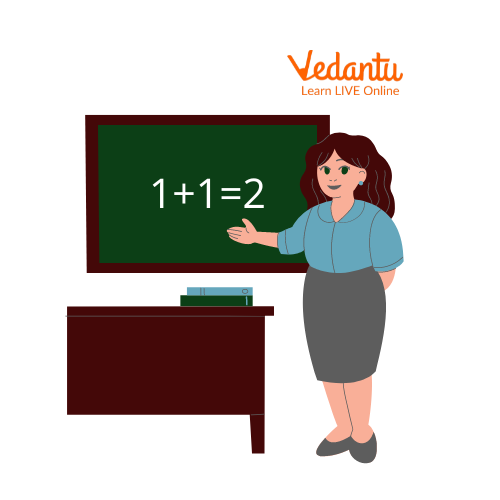Practice Time

1. Find the fraction, if its decimal value is 0.6 and the denominator is 75.

Ans: 3/375

2. The correct decimal representation of the fraction 14/25 is____.

Ans: 0.56

## Let’s Summarise

In this article, we learnt the method of expressing a fraction using a group of common denominators, such as 1/10 and 1/100. The benefit of decimals is that addition, subtraction, and comparison operations are simple because they all have common denominators. Both fractions and decimals are used to describe components of a whole. The concept of a fraction is simpler.

Last updated date: 30th Sep 2023
Total views: 126.6k
Views today: 3.26k

## FAQs on An Easy Way to Convert Fraction to Decimal

1. How to turn a fraction into a decimal and what does 1/3 in decimal form mean?

We must divide the numerator by the denominator in order to convert a fraction to a decimal. We can even utilise a calculator for this operation, if necessary. This will give us the answer in decimal form.

The definition of one-third is one (1) divided into three (3) parts. Therefore, divide it to obtain the decimal equivalent. Three (3) times one (1) equals "0.33333333.," etc. If a decimal is repeated, we can round it to a particular decimal point.

2. What is a fraction above another fraction known as and are fractions and decimals similar?

To divide one fraction by another, we must first flip its numerator and denominator, after which we will multiply the two fractions. The fraction that is reversed is also referred to as the reciprocal or the multiplicative inverse.

Due to the fact that they are both ways of expressing partial numbers, fractions and decimals are equal. Additionally, by dividing by the ratio, fractions can also be expressed as decimals. In terms of tenths, hundredths, thousandths, etc., the decimals can also be expressed as fractions.